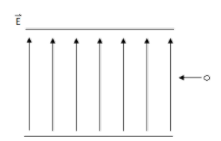# Problem: An electron beam is shot into a region where there is both an electric field pointing upward of magnitude E = 1.6 N/C, and a magnetic field, B, as shown in the figure. While moving through the region the electron beam stays in a straight line with speed v 130 m/s. Randomized Variables:E = 1.6 N/Cv = 130 m/s Part (a) In which direction does the electric force act on the electrons? A. DownwardB. To the left.C. To the right.D. Upward.Part (b) In order to keep the electrons in a straight line, in which direction should the magnetic force act? Part (c) What should the direction of the magnetic field be in order to produce the force in part (b)? Part (d) Express the magnitude of the electric force using the electric field E and the electron charge e. Part (e) Calculate the numerical value of the magnitude of Fe, in newtons.Part (f) Express the magnitude of the magnetic force in terms of the electron charge e, speed v, and B, the magnitude of the magnetic field. Part (g) If the electron is continuing in a straight line, express the magnitude of the magnetic field in terms of v and E. Part (h) Calculate the magnitude of the magnetic field if the electron continues in a straight line, in tesla.

###### FREE Expert Solution

Part (a)

The force acting on the electron is in the opposite direction to the field.

81% (383 ratings)###### Problem Details

An electron beam is shot into a region where there is both an electric field pointing upward of magnitude E = 1.6 N/C, and a magnetic field, B, as shown in the figure. While moving through the region the electron beam stays in a straight line with speed v 130 m/s.Randomized Variables:

E = 1.6 N/C
v = 130 m/s

Part (a) In which direction does the electric force act on the electrons?

A. Downward
B. To the left.
C. To the right.
D. Upward.

Part (b) In order to keep the electrons in a straight line, in which direction should the magnetic force act?

Part (c) What should the direction of the magnetic field be in order to produce the force in part (b)?

Part (d) Express the magnitude of the electric force using the electric field E and the electron charge e.

Part (e) Calculate the numerical value of the magnitude of Fe, in newtons.

Part (f) Express the magnitude of the magnetic force in terms of the electron charge e, speed v, and B, the magnitude of the magnetic field.

Part (g) If the electron is continuing in a straight line, express the magnitude of the magnetic field in terms of v and E.

Part (h) Calculate the magnitude of the magnetic field if the electron continues in a straight line, in tesla.